Setup for 4 Player a Game of Food Chain Magnate

Originally generated on 1/11/2019 7:24:50 AM

Player Setup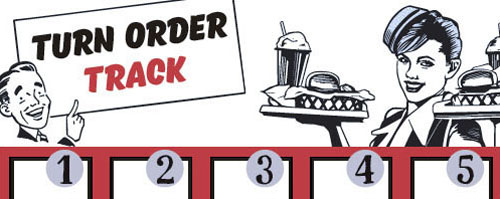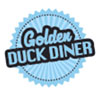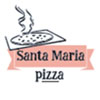Other Setup

Bank starts with \$200.00
Remove these Billboards: #16
Number of 1x Employee cards used = 2

Map (Random)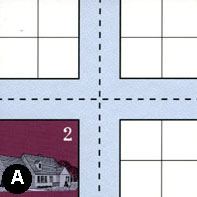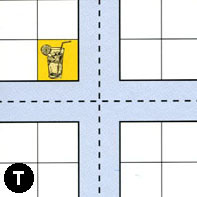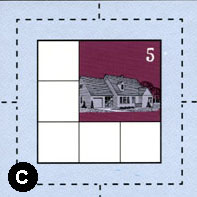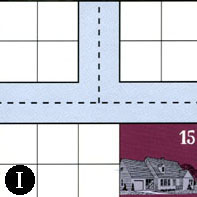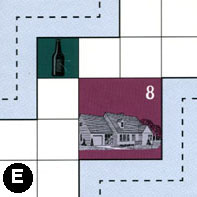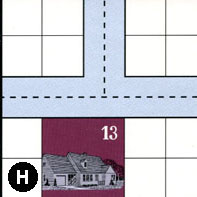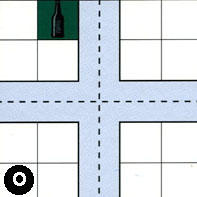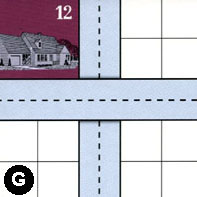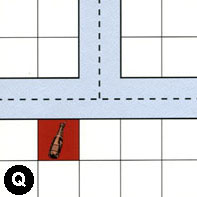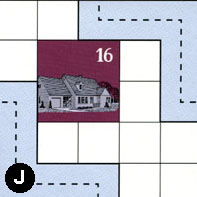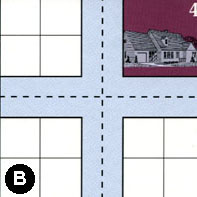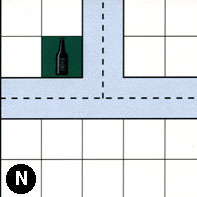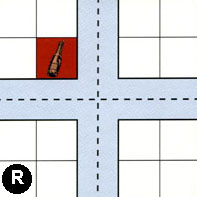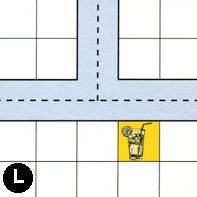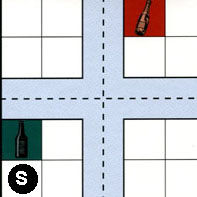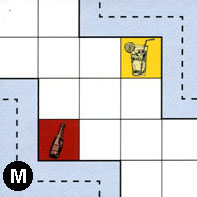Map Stats

Map Option = Random
Total Number of Tiles = 16
Total Number of Starting Houses = 8
Total Number of Beer Spots = 4
Total Number of Soda Spots = 4
Total Number of Lemonade Spots = 3
Total Number of Drink Spots = 11
Number of independent path systems = 3
Number of independent neighborhoods = 17

Path System 1
Size = Medium
Number of Tiles = 14
Number of Paths = 54
Contains Loop(s) = No
Number of Starting Houses = 9
Starting Houses Pct of Map (%) = 112
Number of Beer Spots = 3
Number of Soda Spots = 1
Number of Lemonade Spots = 3
Number of Drink Spots = 7
Drink Spots Pct of Map (%) = 64
Tile Ids = A, B, C, E, G, H, I, J, L, M, N, O, S, T
Paths = AWN, AWE, TWE, CWE, IWN, GNS, NWE, MEN, SWN, BWN, JEN, LWN, SWS, BWE, OWS, HWN, JWS, HNE, EES, AWS, ANS, ANE, TNE, TWN, CNE, OWN, HWE, OWE, GWE, ONE, CWN, INE, IWE, CWS, CNS, ONS, BNE, BNS, NWN, NNE, BWS, SWE, SNE, SNS, LNE, LWE, SES, BES, OES, CES, TWS, TNS, TES, AES What do these mean?
Starting House Ids = 0, 2, 4, 5, 8, 12, 13, 15, 16
Path System 2
Size = Medium
Number of Tiles = 3
Number of Paths = 10
Contains Loop(s) = No
Number of Starting Houses = 1
Starting Houses Pct of Map (%) = 12
Number of Beer Spots = 1
Number of Soda Spots = 2
Number of Lemonade Spots = 0
Number of Drink Spots = 3
Drink Spots Pct of Map (%) = 27
Tile Ids = E, Q, R
Paths = EWN, QWE, RWN, RWE, RNE, QWN, QNE, RNS, RWS, RES What do these mean?
Starting House Ids = 0
Path System 3
Size = Tiny
Number of Tiles = 1
Number of Paths = 1
Contains Loop(s) = No
Number of Starting Houses = 1
Starting Houses Pct of Map (%) = 12
Number of Beer Spots = 0
Number of Soda Spots = 1
Number of Lemonade Spots = 0
Number of Drink Spots = 1
Drink Spots Pct of Map (%) = 9
Tile Ids = M
Paths = MWS What do these mean?
Starting House Ids = 0

Neighborhood 1
Total Size = Small
Number of Total Spaces = 4
Number of Empty Spaces (for new houses & gardens) = 4
Number of Beer Spots = 0
Number of Soda Spots = 0
Number of Lemonade Spots = 0
Number of Drink Spots = 0
Number of Starting Houses = 0
Starting Houses Pct of Map (%) = 0
Tile Ids = A
Neighborhood 2
Total Size = Medium
Number of Total Spaces = 8
Number of Empty Spaces (for new houses & gardens) = 8
Number of Beer Spots = 0
Number of Soda Spots = 0
Number of Lemonade Spots = 0
Number of Drink Spots = 0
Number of Starting Houses = 0
Starting Houses Pct of Map (%) = 0
Tile Ids = A, T
Neighborhood 3
Total Size = Small
Number of Total Spaces = 4
Number of Empty Spaces (for new houses & gardens) = 4
Number of Beer Spots = 0
Number of Soda Spots = 0
Number of Lemonade Spots = 0
Number of Drink Spots = 0
Number of Starting Houses = 0
Starting Houses Pct of Map (%) = 0
Tile Ids = T
Neighborhood 4
Total Size = Small
Number of Total Spaces = 4
Number of Empty Spaces (for new houses & gardens) = 4
Number of Beer Spots = 0
Number of Soda Spots = 0
Number of Lemonade Spots = 0
Number of Drink Spots = 0
Number of Starting Houses = 0
Starting Houses Pct of Map (%) = 0
Tile Ids = I
Neighborhood 5
Total Size = Medium
Number of Total Spaces = 14
Number of Empty Spaces (for new houses & gardens) = 10
Number of Beer Spots = 0
Number of Soda Spots = 0
Number of Lemonade Spots = 0
Number of Drink Spots = 0
Number of Starting Houses = 1
Starting Houses Pct of Map (%) = 12
Tile Ids = G, I
Starting House Ids = 15
Neighborhood 6
Total Size = Medium
Number of Total Spaces = 9
Number of Empty Spaces (for new houses & gardens) = 5
Number of Beer Spots = 0
Number of Soda Spots = 0
Number of Lemonade Spots = 0
Number of Drink Spots = 0
Number of Starting Houses = 1
Starting Houses Pct of Map (%) = 12
Tile Ids = C
Starting House Ids = 5
Neighborhood 7
Total Size = Large
Number of Total Spaces = 78
Number of Empty Spaces (for new houses & gardens) = 59
Number of Beer Spots = 1
Number of Soda Spots = 1
Number of Lemonade Spots = 1
Number of Drink Spots = 3
Number of Starting Houses = 4
Starting Houses Pct of Map (%) = 50
Tile Ids = A, B, E, H, J, L, O, Q, R
Starting House Ids = 2, 4, 8, 16
Neighborhood 8
Total Size = Medium
Number of Total Spaces = 26
Number of Empty Spaces (for new houses & gardens) = 20
Number of Beer Spots = 1
Number of Soda Spots = 0
Number of Lemonade Spots = 1
Number of Drink Spots = 2
Number of Starting Houses = 1
Starting Houses Pct of Map (%) = 12
Tile Ids = A, H, O, T
Starting House Ids = 13
Neighborhood 9
Total Size = Medium
Number of Total Spaces = 12
Number of Empty Spaces (for new houses & gardens) = 8
Number of Beer Spots = 0
Number of Soda Spots = 0
Number of Lemonade Spots = 0
Number of Drink Spots = 0
Number of Starting Houses = 1
Starting Houses Pct of Map (%) = 12
Tile Ids = G, I, O
Starting House Ids = 12
Neighborhood 10
Total Size = Medium
Number of Total Spaces = 16
Number of Empty Spaces (for new houses & gardens) = 16
Number of Beer Spots = 0
Number of Soda Spots = 0
Number of Lemonade Spots = 0
Number of Drink Spots = 0
Number of Starting Houses = 0
Starting Houses Pct of Map (%) = 0
Tile Ids = B, G, N, O
Neighborhood 11
Total Size = Large
Number of Total Spaces = 33
Number of Empty Spaces (for new houses & gardens) = 30
Number of Beer Spots = 0
Number of Soda Spots = 2
Number of Lemonade Spots = 1
Number of Drink Spots = 3
Number of Starting Houses = 0
Starting Houses Pct of Map (%) = 0
Tile Ids = G, M, N, S
Neighborhood 12
Total Size = Small
Number of Total Spaces = 4
Number of Empty Spaces (for new houses & gardens) = 4
Number of Beer Spots = 0
Number of Soda Spots = 0
Number of Lemonade Spots = 0
Number of Drink Spots = 0
Number of Starting Houses = 0
Starting Houses Pct of Map (%) = 0
Tile Ids = Q
Neighborhood 13
Total Size = Medium
Number of Total Spaces = 8
Number of Empty Spaces (for new houses & gardens) = 7
Number of Beer Spots = 0
Number of Soda Spots = 1
Number of Lemonade Spots = 0
Number of Drink Spots = 1
Number of Starting Houses = 0
Starting Houses Pct of Map (%) = 0
Tile Ids = Q, R
Neighborhood 14
Total Size = Medium
Number of Total Spaces = 12
Number of Empty Spaces (for new houses & gardens) = 11
Number of Beer Spots = 1
Number of Soda Spots = 0
Number of Lemonade Spots = 0
Number of Drink Spots = 1
Number of Starting Houses = 0
Starting Houses Pct of Map (%) = 0
Tile Ids = B, L, S
Neighborhood 15
Total Size = Medium
Number of Total Spaces = 12
Number of Empty Spaces (for new houses & gardens) = 11
Number of Beer Spots = 1
Number of Soda Spots = 0
Number of Lemonade Spots = 0
Number of Drink Spots = 1
Number of Starting Houses = 0
Starting Houses Pct of Map (%) = 0
Tile Ids = B, N, S
Neighborhood 16
Total Size = Small
Number of Total Spaces = 4
Number of Empty Spaces (for new houses & gardens) = 4
Number of Beer Spots = 0
Number of Soda Spots = 0
Number of Lemonade Spots = 0
Number of Drink Spots = 0
Number of Starting Houses = 0
Starting Houses Pct of Map (%) = 0
Tile Ids = R
Neighborhood 17
Total Size = Medium
Number of Total Spaces = 8
Number of Empty Spaces (for new houses & gardens) = 8
Number of Beer Spots = 0
Number of Soda Spots = 0
Number of Lemonade Spots = 0
Number of Drink Spots = 0
Number of Starting Houses = 0
Starting Houses Pct of Map (%) = 0
Tile Ids = L, S

v2.1.5